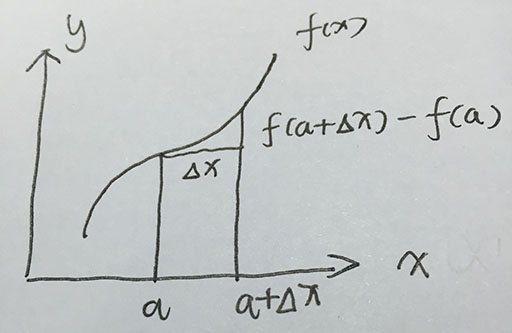机器学习笔记1—泰勒展开式和牛顿法

1.1 泰勒展开式

1.1.1泰勒展开式入门

f(x)=n=0fn(a)n!(xa)n1f(x)=∑n=0∞fn(a)n!(x−a)n（1）

【对于泰勒展开式存在性的一些思考】

1.1.2 泰勒展开式的推导f(a+Δx)f(a)Δx=tanα(2)f(a+Δx)−f(a)Δx=tan⁡α(2)

f(a+Δx)=f(a)+f(a)Δxf(a+Δx)=f(a)+f′(a)Δx

1.2 牛顿法

1、求方程的根（函数比较复杂，没有求根公式）。
2、应用于最优化方法求解无约束问题的最优解（通常是求函数的极大极小值）

1.2.1 求方程的根

(1). 第一步选取初始点，构造一阶泰勒展开式。
x0x0处展开到一阶泰勒公式：f(x)=f(x0)+f(x0)(xx0)f(x)=f(x0)+f′(x0)(x−x0)

x1x1是上式的解：
x1=x0f(x0)f(x0)x1=x0−f′(x0)f(x0)

(2).迭代公式
xn+1=xnf(xn)f(xn)xn+1=xn−f′(xn)f(xn)

1.2.2 求最优化方法（非线性函数的无约束问题）

1、二维情况

(1) 函数极小值的一阶必要条件：若xDx∗∈D是一个极小点，则必有f(x)=0f′(x∗)=0
(2) 函数极小值的二阶必要条件：若xDx∗∈D是一个极小点，则必有f(x)=0f′(x∗)=0&&f′′(x)>0f″(x∗)>0

f(x)=0f(x0)+f′′(x0)(x1x0)=0f′(x)=0⟹f′(x0)+f″(x0)(x1−x0)=0
x1=x0f(x0)f′′(x0)⟹x1=x0−f′(x0)f″(x0)

f(x)=f(xa)+f(xa)(xxa)+12f′′(xa)(xxa)2f(x)=f(xa)+f′(xa)(x−xa)+12f″(xa)(x−xa)2

2、高维度

Xn+1=XnG1kgkXn+1=Xn−Gk−1gk

2015/10/29 first version

f(X),Xf(X),X为n维列向量，表示含有n个变量。

X=x1xnX=(x1⋮xn)

Xk=xk1xknXk=(xk1⋮xkn)

f(X)f(X)XkXk处泰勒二阶展开。

f(Xk)=gk=f(Xk)f′(Xk)=gk=∇f(Xk)

f(X)=f(X)x1f(X)xnn×1∇f(X)=(∂f(X)∂x1⋮∂f(X)∂xn)n×1

f′′(Xk)=Gk=2f(Xk)f″(Xk)=Gk=∇2f(Xk)

2f(X)=2f(X)x212f(X)xnx12f(X)x1xn2f(X)x2nn×n∇2f(X)=(∂2f(X)∂x12⋯∂2f(X)∂x1∂xn⋮⋱⋮∂2f(X)∂xn∂x1⋯∂2f(X)∂xn2)n×n

XXk=x1xk1xnxknn×1X−Xk=(x1−xk1⋮xn−xkn)n×1

f(X)=f(Xk)+gTk(XXk)+12(XXk)TGk(XXk)f(X)=f(Xk)+gkT(X−Xk)+12(X−Xk)TGk(X−Xk)

f(X)f(X)求导时，就不要写成泰勒展开式的形式了，直接写成二维函数那个样子。我把它拆分为三部分。
f(X)1=f(Xk)f(X)1=f(Xk)
f(X)2=f(Xk)(XXk)f(X)2=f′(Xk)(X−Xk)
f(X)3=12f′′(Xk)(XXk)2f(X)3=12f″(Xk)(X−Xk)2
（1） 对f(X)1f(X)1求导，f(Xk)f(Xk)不含有变量XX所以此项导数为0。
（2） 对f(X)2f(X)2求导。
gTkgkT不含有变量XX所以为常数，不需要考虑，这时要求导的部分变成了y=(XXk)y=(X−Xk)。

y=XXk=x1xk1x2xk2x3xk33×1y=X−Xk=(x1−xk1x2−xk2x3−xk3)3×1

yyXX求导：yy为矩阵XX为列向量。属于矩阵对列向量求导的那一类。

yX=y1Xy2Xy3X∂y∂X=(∂y1∂X∂y2∂X∂y3∂X)

y1X=y1x1y1x2y1x3=100∂y1∂X=(∂y1∂x1∂y1∂x2∂y1∂x3)=(100)

y2X=010∂y2∂X=(010)

y3X=001∂y3∂X=(001)

yX=100010001∂y∂X=(100010001)

（3） 对f(X)3f(X)3求导。

f(X)3=f′′(Xk)=Gkf′(X)3=f″(Xk)=Gk

GkGk为非奇异（则矩阵可逆），解这个方程，记其解为Xk+1Xk+1即得牛顿法的迭代公式：
Xk+1=XkG1kgkXk+1=Xk−Gk−1gk

2015/11/3 second version